# Introduction to GDI+ Transformations

Introduction

A transformation is a process that changes graphics objects from one state to another. Rotation, scaling, reflection, translation, and shearing are some examples of transformation. Transformations can be applied not only to graphics shapes, curves, and images, but even to image colors.

In this chapter we will cover the following topics:

• The basics of transformation, including coordinate systems and matrices
• Global, local, and composite transformations
• Transformation functionality provided by the Graphics class
• Transformation concepts such as shearing, rotation, scaling, and translation
• The Matrix and ColorMatrix classes, and their role in transformation
• Matrix operations in image processing, including rotation, translation, shearing, and
scaling
• Color transformation and recoloring
• Text transformation
• Composite transformations and the matrix order

Any drawing process involves a source and a destination. The source of a drawing is the application that created it, and the destination is a display or printer device. For example, the process of drawing a simple rectangle starts with a command telling GDI+ to draw on the screen, followed by GDI+ iterating through multiple steps before it finally renders a rectangle on the screen. In the same way, transformation involves some steps before it actually renders the transformed object on a device. These steps are shown in Figure 10.1, which shows that GDI+ is responsible for converting world coordinates to page coordinates and device coordinates before it can render a transformed object.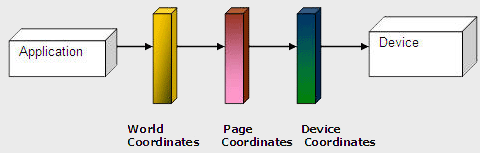Fig 10-1: Steps in the Tranformation Process.

10.1 Coordinate Systems

Before we discuss transformations, we need to understand coordinate systems. GDI+ defines three types of coordinate spaces: world, page, and device. When we ask GDI+ to draw a line from point A (x1, y1) to point B (x2, y2), these points are in the world coordinate system.

Before GDI+ draws a graphics shape on a surface, the shape goes through a few transformation stages (conversions). The first stage converts world coordinates to page coordinates. Page coordinates may or may not be the same as world coordinates, depending on the transformation. The process of converting world coordinates to page coordinates is called world transformation.

The second stage converts page coordinates to device coordinates. Device coordinates represent how a graphics shape will be displayed on a device such as a monitor or printer. The process of converting page coordinates to device coordinates is called page transformation. Figure 10.2 shows the stages of conversion from world coordinates to device coordinates.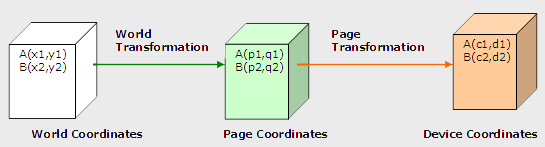Fig 10-2: Transformation Stages.

In GDI+, the default origin of all three coordinate systems is point (0, 0), which is at the upper left corner of the client area. When we draw a line from point A (0, 0) to point B (120, 80), the line starts 0 pixels from the upper left corner in the x-direction and 0 pixels from the upper left corner in the y-direction, and it will end 120 pixels over in the x-direction and 80 pixels down in the y-direction. The line from point A (0, 0) to point B (120, 80) is shown in Figure 10.3.

Drawing this line programmatically is very simple. We must have a Graphics object associated with a surface (a form or a control). We can get a Graphics object in several ways. One way is to accept the implicit object provided by a form's paint event handler; another is to use the CreateGraphics method. Once we have a Graphics object, we call its draw and fill methods to draw and fill graphics objects. Listing 10.1 draws a line from starting point A (0, 0) to ending point B (120, 80). You can add this code to a form's paint event handler.

Listing 10.1:

Drawing a line from point (0, 0) to point (120, 80)
Graphics g = e.Graphics;
Point A =
new Point(0, 0);
Point B =
new Point(120, 80);
g.DrawLine(Pens.Black, A, B);

Figure 10.3 shows the output from Listing 10.1. All three coordinate systems (world, page, and device) draw a line starting from point (0, 0) in the upper left corner of the client area to point (120, 80).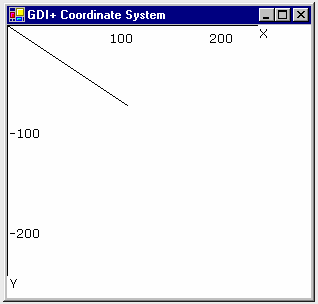Fig 10-3: Drawing a Line from Point(0,0) to (120,80)

Now let's change to the page coordinate system. We draw a line from point A (0, 0) to point B (120, 80), but this time our origin is point (50, 40) instead of the upper left corner. We shift the page coordinates from point (0, 0) to point (50, 40). The TranslateTransform method of the Graphics class does this for us. We will discuss this method in more detail in the discussion that follows. For now, let's try the code in Listing 10.2.

Listing 10.2: Drawing a line from point (0, 0) to point (120, 80) with origin (50, 40)

Graphics g = e.Graphics;
g.TranslateTransform(50, 40);
Point A =
new Point(0, 0);
Point B =
new Point(120, 80);
g.DrawLine(Pens.Black, A, B);

Figure 10.4 shows the output from Listing 10.2. The page coordinate system now starts at point (50, 40), so the line starts at point (0, 0) and ends at point (120, 80). The world coordinates in this case are still (0, 0) and (120, 80), but the page and device coordinates are (50, 40) and (170, 120). The device coordinates in this case are same as the page coordinates because the page unit is in the pixel (default) format.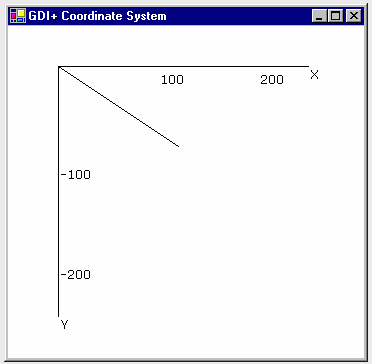Fig 10-4: Drawing a Line from Point(0,0) to (120,80) with the Origin (50,40)

What is the difference between page and device coordinates? Device coordinates determine what we actually see on the screen. They can be represented in many formats, including pixels, millimeters, and inches. If the device coordinates are in pixel format, the page coordinates and device coordinates will be the same (this is typically true for monitors, but not for printers).

The PageUnit property of the Graphics class is of type GraphicsUnit enumeration. In Listing 10.3 we set the PageUnit property to inches. Now graphics objects will be measured in inches, so we need to pass inches instead of pixels. If we draw a line from point (0, 0) to point (2, 1), the line ends 2 inches from the left side and 1 inch from the top of the client area in the page coordinate system. In this case the starting and ending points are (0, 0) and (2, 1) in both world and page coordinates, but the device coordinate system converts them to inches. Hence the starting and ending points in the device coordinate system are (0, 0) and (192, 96), assuming a resolution of 96 dots per inch.

Listing 10.3: Setting the device coordinate system to inches

g.PageUnit = GraphicsUnit.Inch;
g.DrawLine(Pens.Black, 0, 0, 2, 1);

Figure 10.5 shows the output from Listing 10.3. The default width of the pen is 1 page unit, which in this case gives us a pen 1 inch wide.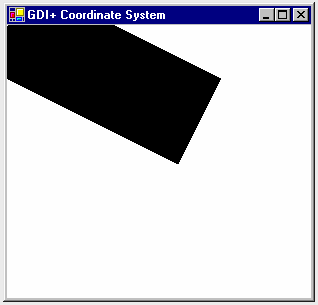Fig 10-5: Drawing with GraphicsUnit.Inch option

Now let's create a new pen with a different width. Listing 10.4 creates a pen that's 1 pixel wide (it does so by dividing the number of pixels we want-in this case 1-by the page resolution, which is given by DpiX). We draw the line again, this time specifying a red color.

Listing 10.4: Using the GraphicsUnit.Inch option with a pixel width

Pen redPen = new Pen(Color.Red, 1/g.DpiX);
g.PageUnit = GraphicsUnit.Inch;
g.DrawLine(Pens.Black, 0, 0, 2, 1);

Figure 10.6 shows the output from Listing 10.4.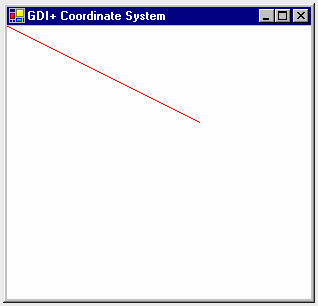Fig 10-6: Drawing with GraphicsUnit.Inch option and a Pixel Width

We can also combine the use of page and device coordinates. In Listing 10.5 we transform page coordinates to 1 inch from the left and 0.5 inch from the top of the upper left corner of the client area. Our new page coordinate system has starting and ending points of (1, 0.5) and (3, 1.5), but the device coordinate system converts them to pixels. Hence the starting and ending points in device coordinates are (96, 48) and (288, 144), assuming a resolution of 96 dots per inch.

Listing 10.5: Combining page and device coordinates

Pen redPen = new Pen(Color.Red, 1/g.DpiX);
g.TranslateTransform(1, 0.5f);
g.PageUnit = GraphicsUnit.Inch;
g.DrawLine(redPen, 0, 0, 2, 1);

Figure 10.7 shows the output from Listing 10.5.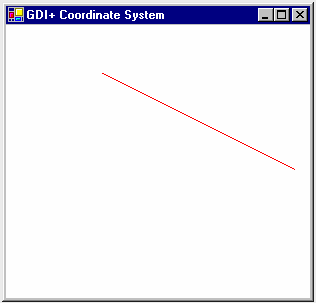Fig 10-7: The output of Listing 10-5.

10.2 Transformation Types

There are many types of transformations.

Translation is a transformation of the xy plane that moves a graphics object toward or away from the origin of the surface in the x- or y-direction. For example, moving an object from point A (x1, y1) to point B (x2, y2) is a translation operation in which an object is being moved (y2 - y1) points in the y-direction.

Rotation moves an object around a fixed angle around the center of the plane.
In the reflection transformation, an object moves to a position in the opposite direction from an axis, along a line perpendicular to the axis. The resulting object is the same distance from the axis as the original point, but in the opposite direction.

Simple transformations, including rotation, scaling, and reflection are called linear transformations. A linear transformation followed by translation is called an affine transformation.

The shearing transformation skews objects based on a shear factor. In the sample applications discussed throughout this chapter, will see how to use these transformations in GDI+.

So far we've looked at only simple transformations. Now let's discuss some more complex transformation-related functionality defined in the .NET Framework library.

What Can You Transform?

You have just seen the basics of transforming lines. We can also transform graphics objects such as points, curves, shapes, images, text, colors, and textures, as well as colors and images used in pens and brushes.

This articles is taken from Chapter 10 "Transformations" of Graphics Programming with GDI+.